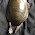## Bi-linear form

Linear map (or transformation) 的意思是，一个由向量空间 U 到 V 的变换，服从：

$T: U \rightarrow V$

$T(v_1 + v_2) = T(v_1) + T(v_2)$

$T(k v) = k T(v)$

Bi-linear map 是 linear map 的推广，由向量空间 U，V 到 W 的变换，使其对 U 和对 V 也是线性变换：

$T: U \times V \rightarrow W$

$T(a v_1 + b v_2, u) = a T(v_1, u) + b T(v_2, u)$

$T(v, a u_1 + b u_2) = a T(v, u_1) + b T(v, u_2)$

## Universal form

Universal form 用来表达某种结构的「最一般形式」，这个 idea 来自 category theory。

In our case，首先考虑两个向量空间之间的 tensor product，⨂：

By definition，那 tensor product 当然是 bi-linear 的。

Bi-linear maps 可以有很多例子，但它们未必是 ⨂ 的模型 (models)。  例如： 两个向量的 inner product、cross product、两个矩阵的 matrix product、两个复数的乘积、 都是 bi-linear 的，但它们都不是 tensor product 的模型，理由是因为它们把双线性变换后的资讯萎缩了 (collapsed)。  但两个矩阵的 Kronecker product 是 tensor product，还有另一个模型是最常见的，利用 dual space V* 来描述。

### Reference:

Guo, Hongyu 2014:  Modern mathematics and applications in computer graphics and vision  这本书很易懂 ，我從這書中抄了一些內容 :

1.2.3.